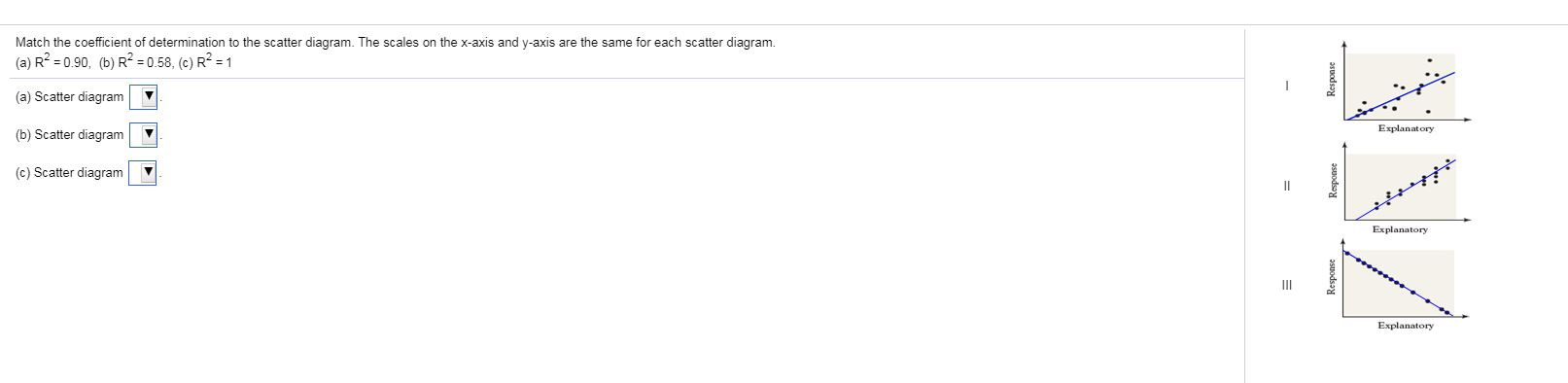# Match the coefficient of determination to the scatter diagram. The scales on the x-axis and y-axis are the same for each scatter diagram(a) R2 0.90, (b) R2 0.58, (c) R2 =1(a) Scatter diagramExplanatory(b) Scatter diagram(c) Scatter diagramExplanatoryIIIExplanatory

Question
154 viewshelp_outlineImage TranscriptioncloseMatch the coefficient of determination to the scatter diagram. The scales on the x-axis and y-axis are the same for each scatter diagram (a) R2 0.90, (b) R2 0.58, (c) R2 =1 (a) Scatter diagram Explanatory (b) Scatter diagram (c) Scatter diagram Explanatory III Explanatory fullscreen
check_circle

Step 1

According to the definition,

R2=r2

Where r is the coefficient of regression.

a)

The graph can be shown below for R-square at 0.90.

The correlation coefficient (r) is 0.9486.

Thus,

Step 2

b)

The graph can be shown below for R-squared at 0.58.

The correlatio...

### Want to see the full answer?

See Solution

#### Want to see this answer and more?

Solutions are written by subject experts who are available 24/7. Questions are typically answered within 1 hour.*

See Solution
*Response times may vary by subject and question.
Tagged in

### Other